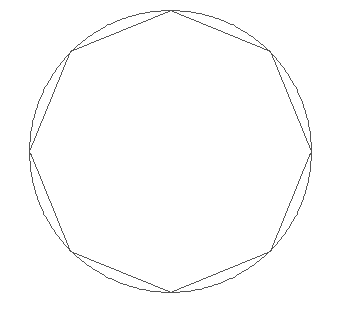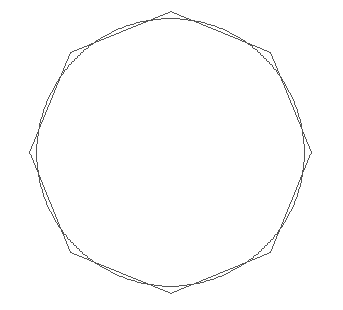Inscribing correction.

The inscribing correction concerns an inaccuracy that can arise when a curved surface is represented by a set of flat segments.  The technique is used in  CPO2D and CPO3D and is illustrated by these figures:The first part shows a circle that is subdivided into 8 segments that all touch the parent circle at their ends, and so are ‘inscribed’.  The second part shows the same subdivision after the ‘inscribing correction’ has been applied by the program, which causes the average distance of each subdivision from the centre of the circle to be equal to the radius of the circle.

An extreme example would be a circle (in CPO2D) that is represented by only 4 straight segments, which would then form a square that touches the circle in 4 places. These segments are 'inscribed' and their mean distance from the centre of the circle is less than the radius of the parent circle. When a uniform charge is put on these 4 segments the potential would be correct at the centres of the segments (as required in the BEM method) but would be incorrect at the centre of the circle and would not have the correct asymptotic form outside the circle.

Most problems are not as extreme as this and the resulting errors in the calculated potentials and fields are usually unimportant, but when the highest accuracy is required they should be corrected by increasing the radius of the electrode.

CPO2D:

The program automatically applies the correction by increasing the radius appropriately.

The correction is not applied if the subdivisions are non-uniform (see note on subdivision of electrodes).

If the correction has been applied and the segments are further subdivided later (see note on subdividing), then the correction continues to be applied in CPO2D.

The user can disable the correction.

CPO3D:

The program applies the correction, if the user requests this, by increasing the radius of the parent electrode (sphere, cylinder or cone) appropriately. The formulas that are used are given note on details of inscribing correction.

The inscribing correction can be applied 'always', sometimes' or 'never'. If the 'sometimes' option is used then the program will apply the correction to all the curved electrodes except those that are given a negative radius, as described in the note on 3D electrode types. The correction can also be applied by hand, before the data is entered, using the formulas given in note on details of inscribing correction.

For technical reasons, the inscribing correction is not allowed in CPO3D if the segments are further subdivided at a later stage in the program (see note on subdivision)